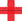top of page

## Video ResourcesIn this video, Luke or Lindsey takes students through a full Stats Medic EFFL lesson.

# Sample Means

##### Learning Targets
• Calculate the mean and standard deviation of the sampling distribution of a sample mean and interpret the standard deviation.

• If appropriate, use a Normal distribution to calculate probabilities involving sample means.

bottom of page